# Reason + ratio - math problems

#### Number of problems found: 83

• 1st drug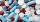First drug pack has an active ingredient ratio of L1: L2 = 2: 1 Second drug pack have a ratio of active ingredients L1: L2 = 1: 3 In which ratio do we have to mix the two packages so that the ratio of substances L1: L2 = 1: 2?
• Chocolate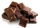I have a box of chocolate - white, milk and dark. The ratio of white to milk with dark is 3: 4. The ratio of white and milk to dark is 17: 4. Calculate what is the ratio between white, milk, dark chocolate.
• Family and age ratiosAge of father and son are in the ratio 10:3; age of father and daughter are in the ratio 5:2. How old is father and son, if daughter is 20 years old? In what ratio is age sister and brother?
• Cuboid and ratioCuboid has dimensions in ratio 1:2:6 and the surface area of the cuboid is 1000 dm2. Calculate the volume of the cuboid.
• BW-BS balls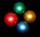Adam has a full box of balls that are large or small, black or white. Ratio of large and small balls is 5:3. Within the large balls the ratio of the black to white is 1:2 and between small balls the ratio of the black to white is 1:8 What is the ratio of
• Ratio of two unknown numbersTwo numbers are given. Their sum is 30. We calculate one-sixth of a larger number and add to both numbers. So we get new numbers whose ratio is 5:7. Which two numbers were given?
• The classroom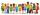In the seventh class the number of boys and girls are at a ratio of 4:5. Boys are 16. How many total students are in the classroom?
• Three numbers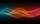The sum of the three numbers is 287. These numbers are in a ratio of 3: 7: 1/4 Define these numbers
• Gold inheritance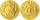The king divided the inheritance with his three sons in the ratio of 7: 6: 4. Two of them received 286,000 gold. How much each of the sons got.
• Oranges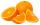Mother divided her three children's oranges in a ratio of 6:5:4. Two children gave 45 oranges. How many oranges were there?
• Inflation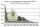What is better for people (employees)? ?
• Division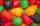Three siblings Helena, Oliver and George split the bag with candies on merit in the ratio 6:1:4. How many candies should each get if in bag were 88?
• Final exam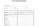At the final exam, the student answers from three areas, which are evaluated in a ratio of 1: 2: 2. What grade will John receive if he answered as follows: 3,1,2.
• Isosceles triangleThe perimeter of an isosceles triangle is 112 cm. The length of the arm to the length of the base is at ratio 5:6. Find the triangle area.
• Collection of stampsJano, Rado, and Fero have created a collection of stamps in a ratio of 5: 6: 9. Two of them had 429 stamps together. How many stamps did their shared collection have?
• Chicken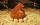I threw three chicken for a handful of grains and I noticed that it eat in a ratio of 8:7:6 and two of them grabbed 156 grains. How many grains have fought that chicken?
• Tea blendTea blends are maked from two kinds of tea. In standard tea mixture are two teas in the ratio 1:3 and 40 g costs 42 CZK. In the premium tea mixture are weighing two teas in the ratio 1:1 and 50 grams costs 60 CZK. How much cost 10 grams of more expensive
• Three friendsThree friends had balls in ratio 2: 7: 4 at the start of the game. Could they have the same number of balls at the end of the game? Write 0, if not, or write the minimum number of balls they had together.
• Lottery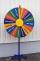The lottery is 60000 elk in which 6200 wins. What is the probability that the purchase of 12 elks won nothing?
• Book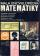To number pages of the thick book was used 4201 digits. How many pages has this book?

Do you have an interesting mathematical word problem that you can't solve it? Submit a math problem, and we can try to solve it.

We will send a solution to your e-mail address. Solved examples are also published here. Please enter the e-mail correctly and check whether you don't have a full mailbox.

Please do not submit problems from current active competitions such as Mathematical Olympiad, correspondence seminars etc...

Check out our ratio calculator.